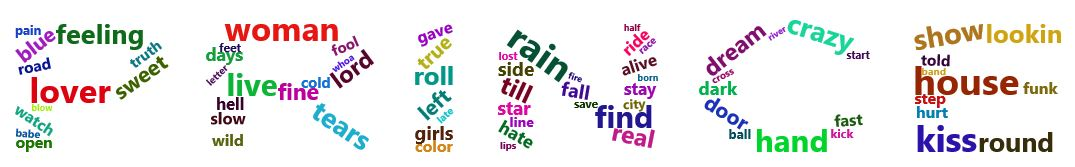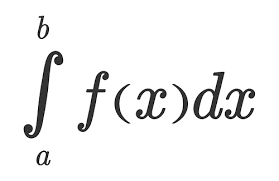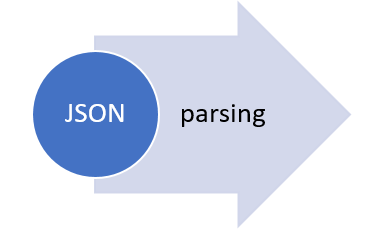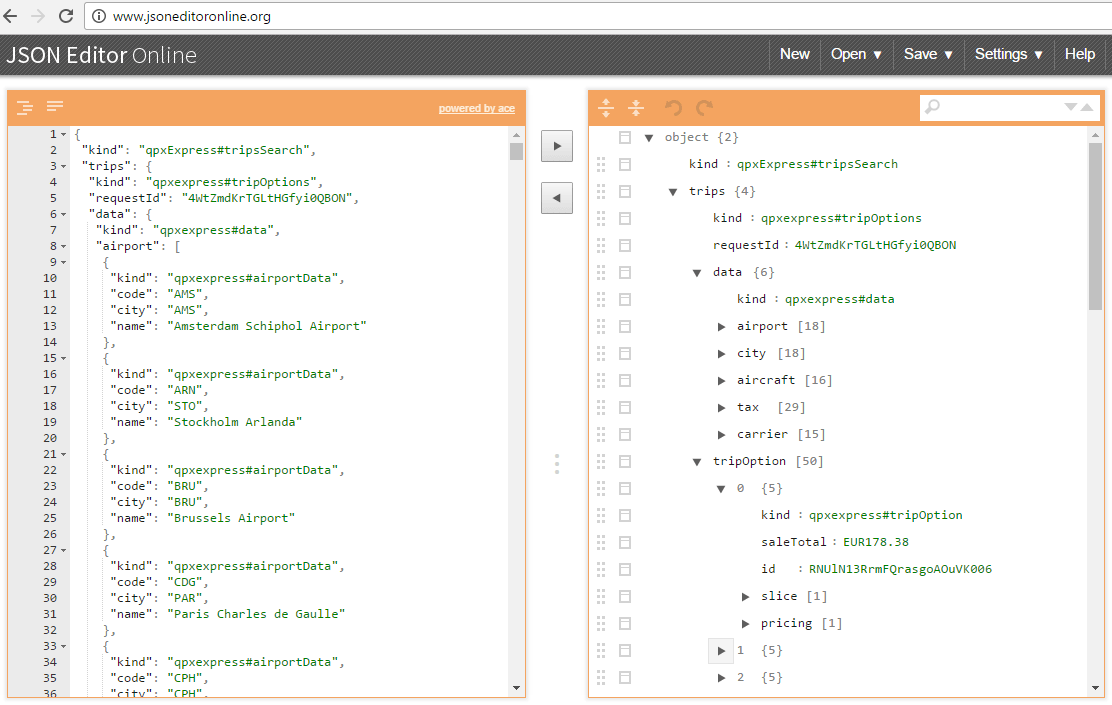## Tutorial: Cleaning and filtering data from Qualtrics surveys, and creating new variables from existing data

Hi fellow R users (and Qualtrics users),

As many Qualtrics surveys produce really similar output datasets, I created a tutorial with the most common steps to clean and filter data from datasets directly downloaded from Qualtrics.

You will also find some useful codes to handle data such as creating new variables in the dataframe from existing variables with functions and logical operators.

The tutorial is presented in the format of a downloadable R code with  explanations and annotations of each step. You will also find a raw Qualtrics dataset to work with.

This dataset comes from a Qualtrics survey with an experiment format (control and treatment conditions), but the codes can be applicable to non-experimental datasets as well, as many cleaning steps are the same.

## A single loop is not enough. A collection of hello world control structuresAs the post on “hello world” functions has been quite appreciated by the R community, here follows the second round of functions for wannabe R programmer.

```# If else statement:
# See the code syntax below for if else statement
x=10
if(x>1){
print(“x is greater than 1”)
}else{
print(“x is less than 1”)
}

# See the code below for nested if else statement

x=10
if(x>1 & x<7){
print(“x is between 1 and 7”)} else if(x>8 & x< 15){
print(“x is between 8 and 15”)
}

# For loops:
# Below code shows for loop implementation
x = c(1,2,3,4,5)
for(i in 1:5){
print(x[i])
}

# While loop :
# Below code shows while loop in R
x = 2.987
while(x <= 4.987) {
x = x + 0.987
print(c(x,x-2,x-1))
}

# Repeat Loop:
# The repeat loop is an infinite loop and used in association with a break statement.

# Below code shows repeat loop:
a = 1
repeat{
print(a)
a = a+1
if (a > 4) {
break
}
}

# Break statement:
# A break statement is used in a loop to stop the iterations and flow the control outside of the loop.

#Below code shows break statement:
x = 1:10
for (i in x){
if (i == 6){
break
}
print(i)
}

# Next statement:
# Next statement enables to skip the current iteration of a loop without terminating it.

#Below code shows next statement
x = 1: 4
for (i in x) {
if (i == 2){
next
}
print(i)
}

# function

words = c(“R”, “datascience”, “machinelearning”,”algorithms”,”AI”)
words.names = function(x) {
for(name in x){
print(name)
}
}

words.names(words) # Calling the function

# extract the elements above the main diagonal of a (square) matrix
# example of a correlation matrix

cor_matrix <- matrix(c(1, -0.25, 0.89, -0.25, 1, -0.54, 0.89, -0.54, 1), 3,3)
rownames(cor_matrix) <- c(“A”,”B”,”C”)
colnames(cor_matrix) <- c(“A”,”B”,”C”)
cor_matrix

rho <- list()
name <- colnames(cor_matrix)
var1 <- list()
var2 <- list()
for (i in 1:ncol(cor_matrix)){
for (j in 1:ncol(cor_matrix)){
if (i != j & i<j){
rho <- c(rho,cor_matrix[i,j])
var1 <- c(var1, name[i])
var2 <- c(var2, name[j])
}
}
}

d <- data.frame(var1=as.character(var1), var2=as.character(var2), rho=as.numeric(rho))
d

var1 var2 rho
1 A B -0.25
2 A C 0.89
3 B C -0.54

```

As programming is the best way to learn and think, have fun programming awesome functions!

This post is also shared in R-bloggers and LinkedIn

## Lyric Analysis with NLP and Machine Learning using R: Part One – Text Mining

June 22
By Debbie Liske

This is Part One of a three part tutorial series originally published on the DataCamp online learning platform in which you will use R to perform a variety of analytic tasks on a case study of musical lyrics by the legendary artist, Prince. The three tutorials cover the following:

Musical lyrics may represent an artist’s perspective, but popular songs reveal what society wants to hear. Lyric analysis is no easy task. Because it is often structured so differently than prose, it requires caution with assumptions and a uniquely discriminant choice of analytic techniques. Musical lyrics permeate our lives and influence our thoughts with subtle ubiquity. The concept of Predictive Lyrics is beginning to buzz and is more prevalent as a subject of research papers and graduate theses. This case study will just touch on a few pieces of this emerging subject.Prince: The Artist

To celebrate the inspiring and diverse body of work left behind by Prince, you will explore the sometimes obvious, but often hidden, messages in his lyrics. However, you don’t have to like Prince’s music to appreciate the influence he had on the development of many genres globally. Rolling Stone magazine listed Prince as the 18th best songwriter of all time, just behind the likes of Bob Dylan, John Lennon, Paul Simon, Joni Mitchell and Stevie Wonder. Lyric analysis is slowly finding its way into data science communities as the possibility of predicting “Hit Songs” approaches reality.

Prince was a man bursting with music – a wildly prolific songwriter, a virtuoso on guitars, keyboards and drums and a master architect of funk, rock, R&B and pop, even as his music defied genres. – Jon Pareles (NY Times)
In this tutorial, Part One of the series, you’ll utilize text mining techniques on a set of lyrics using the tidy text framework. Tidy datasets have a specific structure in which each variable is a column, each observation is a row, and each type of observational unit is a table. After cleaning and conditioning the dataset, you will create descriptive statistics and exploratory visualizations while looking at different aspects of Prince’s lyrics.

Check out the article here!(reprint by permission of DataCamp online learning platform)

## R as learning tool: solving integralsIntegrals are so easy only math teachers could make them difficult.When I was in high school I really disliked math and, with hindsight, I would say it was just because of the the prehistoric teaching tools (when I saw this video I thought I’m not alone). I strongly believe that interaction CAUSES learning (I’m using “causes” here on purpose being quite aware of the difference between correlation and causation), practice should come before theory and imagination is not a skill you, as a teacher, could assume in your students. Here follows a short and simple practical explanation of integrals. The only math-thing I will write here is the following: f(x) = x + 7. From now on everything will be coded in R. So, first of all, what is a function? Instead of using the complex math philosophy let’s just look at it with a programming eye: it is a tool that takes something in input and returns something else as output. For example, if we use the previous tool with 2 as an input we get a 9. Easy peasy. Let’s look at the code:
```# here we create the tool (called "f")
# it just takes some inputs and add it to 7
f <- function(x){x+7}

# if we apply it to 2 it returns a 9
f(2)
9

```
Then the second question comes by itself. What is an integral? Even simpler, it is just the sum of this tool applied to many inputs in a range. Quite complicated, let’s make it simpler with code:
```# first we create the range of inputs
# basically x values go from 4 to 6
# with a very very small step (0.01)
# seq stands for sequence(start, end, step)
```

```x <- seq(4, 6, 0.01)
x
4.00 4.01 4.02 4.03 4.04 4.05 4.06 4.07...

x
4

x
4.01
```
As you see, x has many values and each of them is indexed so it’s easy to find, e.g. the first element is 4 (x). Now that we have many x values (201) within the interval from 4 to 6, we compute the integral.
```# since we said that the integral is
# just a sum, let's call it IntSum and
# set it to the start value of 0
# in this way it will work as an accumulator
```
```IntSum = 0
```
Differently from the theory in which the calculation of the integral produces a new non-sense formula (just kidding, but this seems to be what math teachers are supposed to explain), the integral does produce an output, i.e. a number. We find this number by summing the output of each input value we get from the tool (e.g. 4+7, 4.01+7, 4.02+7, etc) multiplied by the step between one value and the following (e.g. 4.01-4, 4.02-4.01, 4.03-4.02, etc). Let’s clarify this, look down here:
```# for each value of x
for(i in 2:201){

# we do a very simple thing:
# we cumulate with a sum
# the output value of the function f
# multiplied by each steps difference

IntSum = IntSum + f(x[i])*(x[i]-x[i-1])

# So for example,
# with the first and second x values the numbers will be:
#0.1101 = 0 + (4.01 + 7)*(4.01 - 4)

# with the second and third:
#0.2203 = 0.1101 + (4.02 + 7)*(4.02 - 4.01)

# with the third and fourth:
#0.3306 = 0.2203 + (4.03 + 7)*(4.03 - 4.02)

# and so on... with the sum (integral) growing and growing
# up until the last value
}

IntSum
24.01
```
Done! We have the integral but let’s have a look to the visualization of this because it can be represented and made crystal clear. Let’s add a short line of code to serve the purpose of saving the single number added to the sum each time. The reason why we decide to call it “bin” instead of, for example, “many_sum” will be clear in a moment.
```# we need to store 201 calculation and we
# simply do what we did for IntSum but 201 times
bin = rep(0, 201)
bin
0 0 0 0 0 0 0 0 0 0 0 0 ...
```
Basically, we created a sort of memory to host each of the calculation as you see down here:
```for (i in 2:201){

# the sum as earlier
IntSum = IntSum + f(x[i])*(x[i]-x[i-1])

# overwrite each zero with each number
bin[i] = f(x[i])*(x[i]-x[i-1])
}

IntSum
24.01

bin
0.0000 0.1101 0.1102 0.1103 0.1104 0.1105 ..

sum(bin)
24.01
```
Now if you look at the plot below you get the whole story: each bin is a tiny bar with a very small area and is the smallest part of the integral (i.e. the sum of all the bins).
```# plotting them all
barplot(bin, names.arg=x)
```This tells you a lot about the purpose of integral and the possibility of calculating areas of curvy surfaces. To have an idea of this just change the function f with, let’s say, sin(x) or log(x). What is happening? And what if you increase/decrease the number of bins? Have fun replicating the code changing some numbers and functions. Integrals should be clearer in the end. That’s all folks! #R #rstats #maRche #Rbloggers

## “Print hello”​ is not enough. A collection of Hello world functions.I guess I wrote my R “hello world!” function 7 or 8 years ago while approaching R for the first time. And it is too little to illustrate the basic syntax of a programming language for a working program to a wannabe R programmer. Thus, here follows a collection of basic functions that may help a bit more than the famed piece of code.

```######################################################
############### Hello world functions ################
######################################################
##################################
# General info
fun <- function( arguments ) { body }

##################################
x+y
}

----------------------------------

foo.above <- function(x){
x[x>10]
}

foo.above(1:100)

----------------------------------

foo.above_n <- function(x,n){
x[x>n]
}

foo.above_n(1:20, 12)

----------------------------------

foo = seq(1, 100, by=2)
foo.squared = NULL

for (i in 1:50 ) {
foo.squared[i] = foo[i]^2
}

foo.squared

----------------------------------

a <- c(1,6,7,8,8,9,2)

s <- 0
for (i in 1:length(a)){
s <- s + a[[i]]
}
s

----------------------------------

a <- c(1,6,7,8,8,9,2,100)

s <- 0
i <- 1
while (i <= length(a)){
s <- s + a[[i]]
i <- i+1
}
s

----------------------------------

FunSum <- function(a){
s <- 0
i <- 1
while (i <= length(a)){
s <- s + a[[i]]
i <- i+1
}
print(s)
}

FunSum(a)

-----------------------------------

SumInt <- function(n){
s <- 0
for (i in 1:n){
s <- s + i
}
print(s)
}

SumInt(14)

-----------------------------------
# find the maximum
# right to left assignment
x <- c(3, 9, 7, 2)

# trick: it is necessary to use a temporary variable to allow the comparison by pairs of
# each number of the sequence, i.e. the process of comparison is incremental: each time
# a bigger number compared to the previous in the sequence is found, it is assigned as the
# temporary maximum
# Since the process has to start somewhere, the first (temporary) maximum is assigned to be
# the first number of the sequence

max <- x
for(i in x){
tmpmax = i
if(tmpmax > max){
max = tmpmax
}
}

max

x <- c(-20, -14, 6, 2)
x <- c(-2, -24, -14, -7)

min <- x
for(i in x){
tmpmin = i
if(tmpmin < min){
min = tmpmin
}
}

min

----------------------------------
# n is the nth Fibonacci number
# temp is the temporary variable

Fibonacci <- function(n){
a <- 0
b <- 1
for(i in 1:n){
temp <- b
b <- a
a <- a + temp
}
return(a)
}

Fibonacci(13)

----------------------------------
# R available factorial function
factorial(5)

# recursive function: ff
ff <- function(x) {
if(x<=0) {
return(1)
} else {
return(x*ff(x-1)) # function uses the fact it knows its own name to call itself
}
}
ff(5)

----------------------------------

say_hello_to <- function(name){
paste("Hello", name)
}
say_hello_to("Roberto")

----------------------------------

foo.colmean <- function(y){
nc <- ncol(y)
means <- numeric(nc)
for(i in 1:nc){
means[i] <- mean(y[,i])
}
means
}

foo.colmean(airquality)

----------------------------------

foo.colmean <- function(y, removeNA=FALSE){
nc <- ncol(y)
means <- numeric(nc)
for(i in 1:nc){
means[i] <- mean(y[,i], na.rm=removeNA)
}
means
}

foo.colmean(airquality, TRUE)

----------------------------------

foo.contingency <- function(x,y){
nc <- ncol(x)
out <- list()
for (i in 1:nc){
out[[i]] <- table(y, x[,i])
}
names(out) <- names(x)
out
}

set.seed(123)
v1 <- sample(c(rep("a", 5), rep("b", 15), rep("c", 20)))
v2 <- sample(c(rep("d", 15), rep("e", 20), rep("f", 5)))
v3 <- sample(c(rep("g", 10), rep("h", 10), rep("k", 20)))

data <- data.frame(v1, v2, v3)

foo.contingency(data,v3)
```
That's all folks! #R #rstats #maRche #Rbloggers This post is also shared in LinkedIn and www.r-bloggers.com

## Web data acquisition: from database to dataframe for data analysis and visualization (Part 4)

The previous post described how the deeply nested JSON data on fligths were parsed and stored in an R-friendly database structure. However, looking into the data, the information is not yet ready for statistical analysis and visualization and some further processing is necessary before extracting insights and producing nice plots. In the parsed batch, it is clearly visible the redundant structure of the data with the flight id repeted for each segment of each flight. This is also confirmed with the following simple check as the rows of the dataframe are more than the unique counts of the elements in the id column.
```dim(data_items)
 397  15

length(unique(data_items\$id))
201

# real time changes of data could produce different results
```
This implies that the information of each segment of each flight has to be aggregated and merged in a dataset as single observations of a statistical analysis between, for example, price and distance. First, a unique primary key for each observation has to be used as reference variable to uniquely identify each element of the dataset.
```library(plyr) # sql like functions
library(readr) # parse numbers from strings

data_items <- data.frame(data_items)

# id (primary key)
data <- data.frame(unique(data_items\$id))
colnames(data) <- c('id')

# n° of segment
n_segment <- aggregate(data_items['segment.id'], by=data_items['id'], length)
data <- join(data, n_segment, by='id', type='left', match='first') # sql left join
# mileage
mileage <- aggregate(data_items['segment.leg.mileage'], by=data_items['id'], sum)
data <- join(data, mileage, by='id', type='left', match='first') # sql left join
# price
price <- data.frame('id'=data_items\$id, 'price'=parse_number(data_items\$saleTotal))
data <- join(data, price, by='id', type='left', match='first') # sql left join
# dataframe
colnames(data) <- c('id','segment', 'mileage', 'price')

```
The aggregation of mileage and price using the unique primary key allows to set up a dataframe ready for statistical analysis and data visualization. Current data tells us that there is a maximum of three segments in the connection between FCO and LHR with a minimum price of around EUR 122 and a median around EUR 600.
```# descriptive statistics
summary(data)

# histogram price & distance
g1 <- ggplot(data, aes(x=price)) +
geom_histogram(bins = 50) +
ylab("Distribution of the Price (EUR)") +
xlab("Price (EUR)")

g2 <- ggplot(data, aes(x=mileage)) +
geom_histogram(bins = 50) +
ylab("Distribution of the Distance") +
xlab("Distance (miles)")

grid.arrange(g1, g2)
# price - distance relationship
s0 <- ggplot(data = data, aes(x = mileage, y = price)) +
geom_smooth(method = "lm", se=FALSE, color="black") +
geom_point() + labs(x = "Distance in miles", y = "Price (EUR)")
s0 <- ggMarginal(s0, type = "histogram", binwidth = 30)
s0

```
Of course, plenty of other analysis and graphical representations using flights features are possible given the large set of variables available in QPX Express API and the availability of data in real time.To conclude the 4-step (flight) trip from data acquisition to data analysis, let's recap the most important concepts described in each of the post: 1) Client-Server connection 2) POST request in R 3) Data parsing and structuring 4) Data analysis and visualizationThat's all folks! #R #rstats #maRche #json #curl #qpxexpress #Rbloggers This post is also shared in www.r-bloggers.com

## Web data acquisition: parsing json objects with tidyjson (Part 3)The collection of example flight data in json format available in part 2, described the libraries and the structure of the POST request necessary to collect data in a json object. Despite the process generated and transferred locally a proper response, the data collected were neither in a suitable structure for data analysis nor immediately readable. They appears as just a long string of information nested and separated according to the JavaScript object notation syntax. Thus, to visualize the deeply nested json object and make it human readable and understandable for further processing, the json content could be copied and pasted in a common online parser. The tool allows to select each node of the tree and observe the data structure up to the variables and data of interest for the statistical analysis. The bulk of the relevant information for the purpose of the analysis on flight prices are hidden in the tripOption node as shown in the following figure (only 50 flight solutions were requested).However, looking deeply into the object, several other elements are provided as the distance in mile, the segment, the duration, the carrier, etc. The R parser to transform the json structure in a usable dataframe requires the dplyr library for using the pipe operator (%>%) to streamline the code and make the parser more readable. Nevertheless, the library actually wrangling through the lines is tidyjson and its powerful functions:
• enter_object: enters and dives into a data object;
• gather_array: stacks a JSON array;
• spread_values: creates new columns from values assigning specific type (e.g. jstring, jnumber).